# clustered dotplot for single-cell RNAseq

Dotplot is a nice way to visualize scRNAseq expression data across clusters. It gives information (by color) for the average expression level across cells within the cluster and the percentage (by size of the dot) of the cells express that gene within the cluster.

Seurat has a nice function for that. However, it can not do the clustering for the rows and columns. David McGaughey has written a blog post using ggplot2 and ggtree from Guangchuang Yu. It looks great!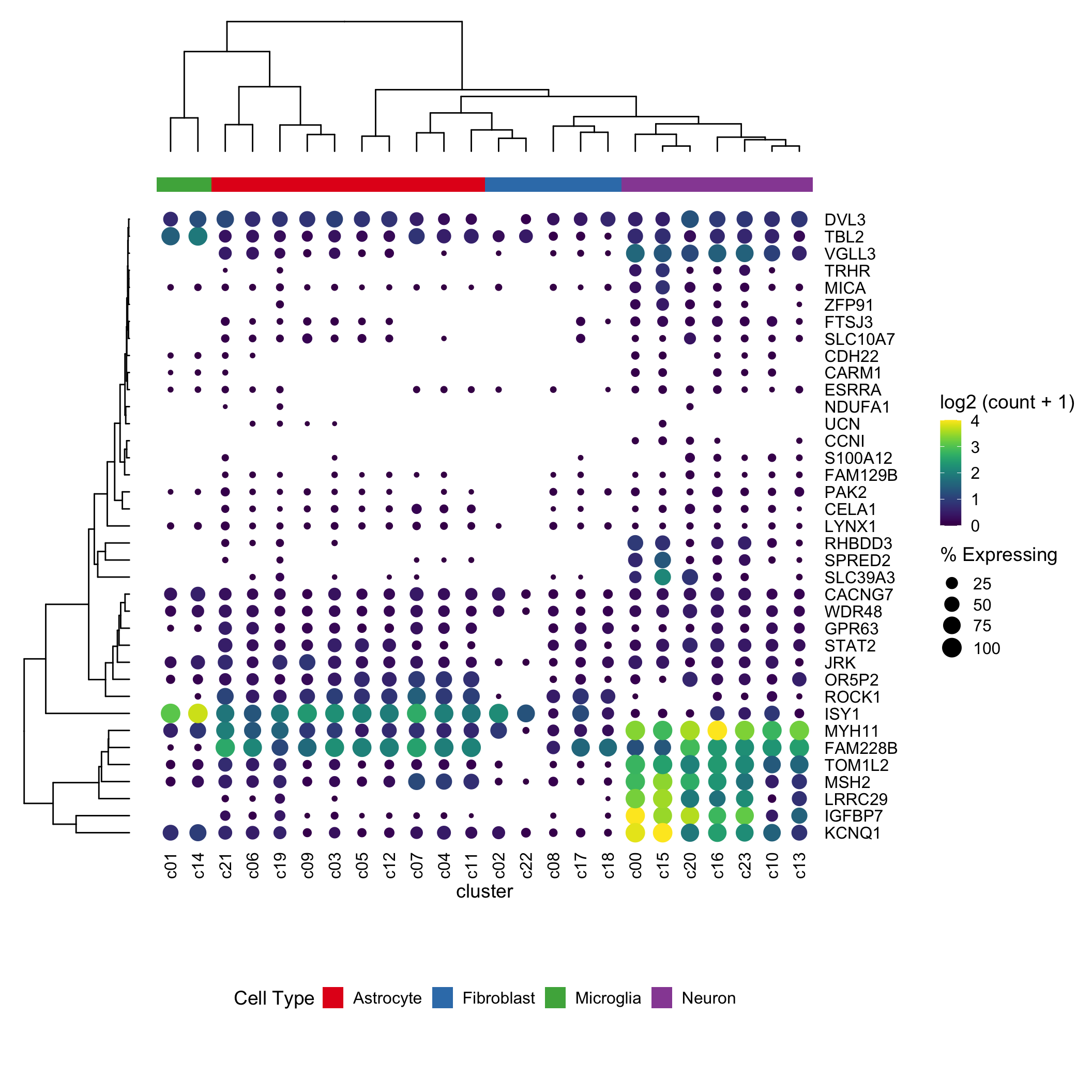In this post, I am trying to reproduce the same effect using ComplexHeatmap

library(Seurat)
library(tidyverse)
library(presto)
library(ComplexHeatmap)
library(circlize)
pbmc <- CreateSeuratObject(counts = pbmc.data, project = "pbmc3k", min.cells = 3, min.features = 200)

### standard processing

pbmc[["percent.mt"]] <- PercentageFeatureSet(pbmc, pattern = "^MT-")

## ScaleData uses top variable genes only
pbmc<- pbmc %>%
NormalizeData(normalization.method = "LogNormalize", scale.factor = 10000) %>%
FindVariableFeatures(selection.method = "vst", nfeatures = 2000) %>%
ScaleData() %>%
RunPCA() %>%
FindNeighbors(dims = 1:10) %>%
FindClusters(resolution = 0.5) %>%
RunUMAP(dims = 1:10)
## Modularity Optimizer version 1.3.0 by Ludo Waltman and Nees Jan van Eck
##
## Number of nodes: 2700
## Number of edges: 97958
##
## Running Louvain algorithm...
## Maximum modularity in 10 random starts: 0.8717
## Number of communities: 9
## Elapsed time: 0 seconds
DimPlot(pbmc, reduction = "umap")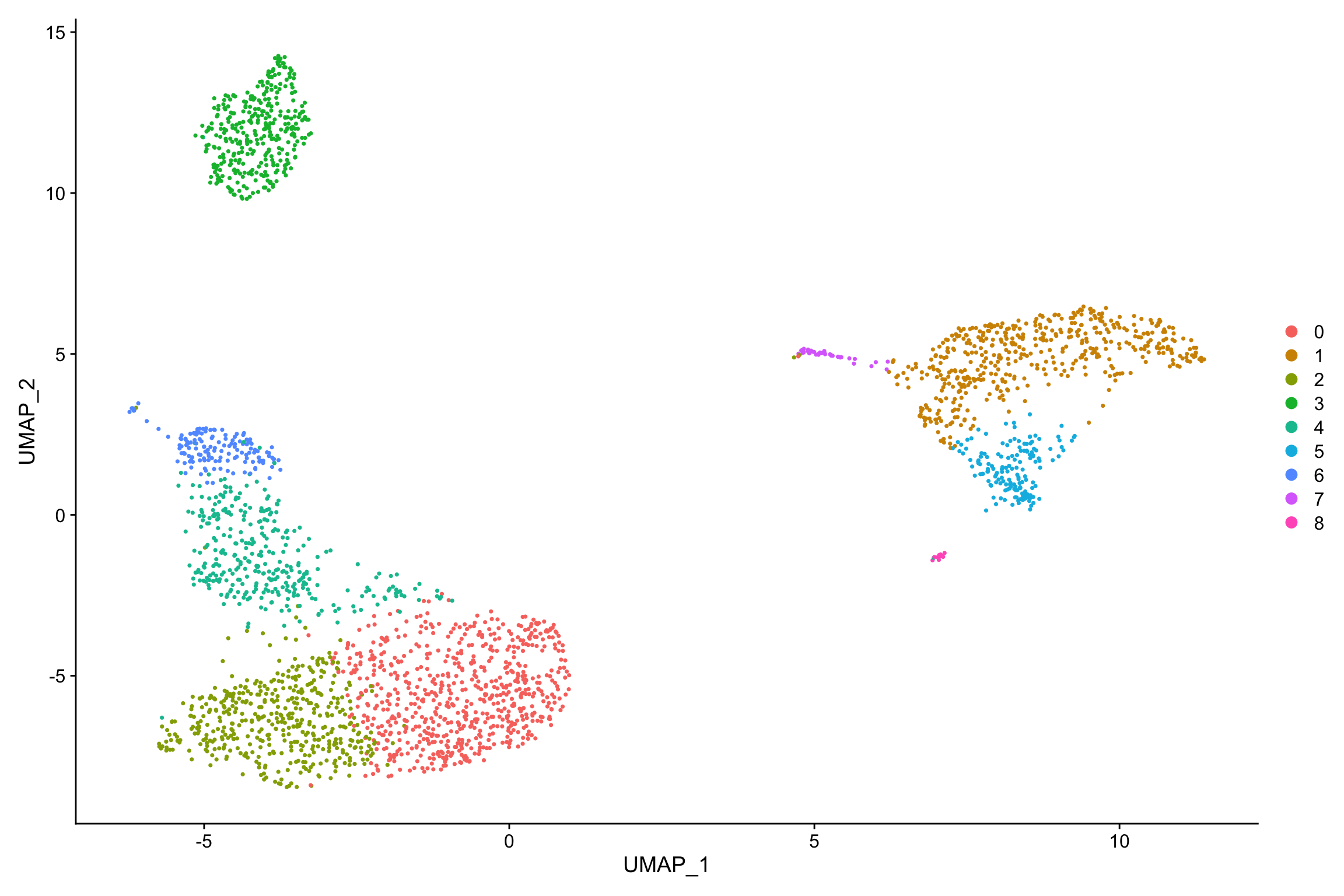new.cluster.ids <- c("Naive CD4 T", "CD14+ Mono", "Memory CD4 T", "B", "CD8 T", "FCGR3A+ Mono",
"NK", "DC", "Platelet")
names(new.cluster.ids) <- levels(pbmc)
pbmc <- RenameIdents(pbmc, new.cluster.ids)
DimPlot(pbmc, reduction = "umap", label = TRUE, pt.size = 0.5) + NoLegend()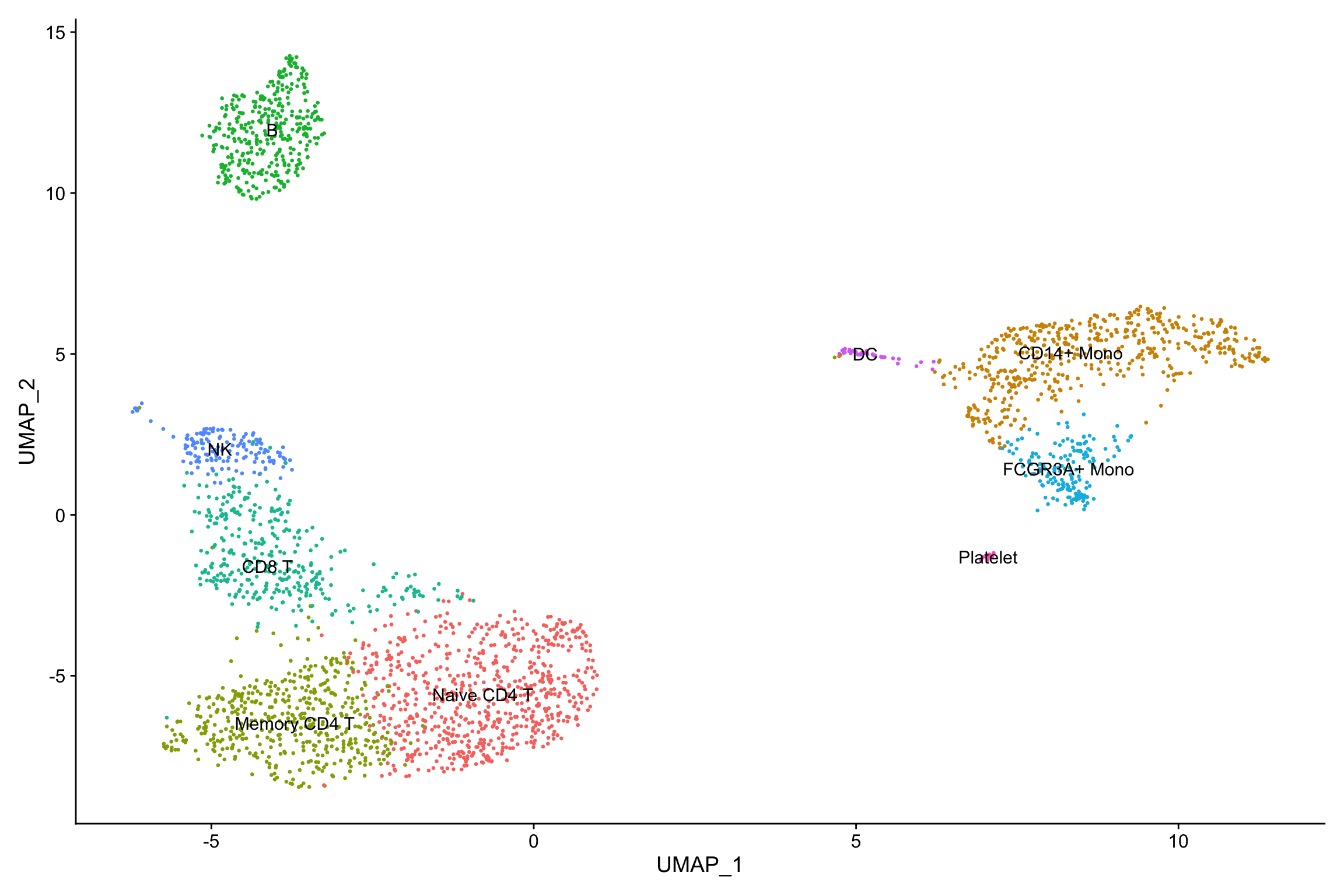### find marker genes

markers<- presto::wilcoxauc(pbmc, 'seurat_clusters', assay = 'data')
markers<- top_markers(markers, n = 10, auc_min = 0.5, pct_in_min = 20, pct_out_max = 20)

markers
## # A tibble: 10 x 10
##     rank 0      1    2    3      4   5         6   7     8
##    <int> <chr>    <chr>  <chr>  <chr>    <chr> <chr>       <chr> <chr>   <chr>
##  1     1 CCR7     S100A8 AQP3   CD79A    GZMA  FCGR3A      GZMB  FCER1A  PPBP
##  2     2 PIK3IP1  FCN1   TRAT1  CD79B    CST7  IFITM3      PRF1  CLEC10A NRGN
##  3     3 PRKCQ-A… LGALS2 SPOCK2 MS4A1    GZMK  RP11-290F2… GNLY  HLA-DQ… PF4
##  4     4 LEF1     CFD    CD27   HLA-DQA1 LYAR  CFD         FGFB… CPVL    SDPR
##  5     5 TCF7     GRN    TRADD  HLA-DQB1 GZMM  MS4A7       CST7  HLA-DMB GNG11
##  6     6 CD27     MS4A6A CD3G   TCL1A    CD8A  CD68        GZMA  CD33    SPARC
##  7     7 MAL      AP1S2  RGCC   LINC009… KLRG1 SPI1        FCGR… CTSH    RGS18
##  8     8 RGCC     CD14   CD40LG HLA-DMA  PRF1  RHOC        SPON2 RNASE6  HIST1H…
##  9     9 CD3G     CD68   LAT    VPREB3   GZMH  HCK         CCL4  KLF4    TPM4
## 10    10 LDLRAP1  LGALS3 FLT3LG HLA-DQA2 HOPX  IFI30       APMAP RNF130  GP9
all_markers<- markers %>%
select(-rank) %>%
unclass() %>%
stack() %>%
pull(values) %>%
unique() %>%
.[!is.na(.)]

Seurat’s dot plot

p<- DotPlot(object = pbmc, features = all_markers)
p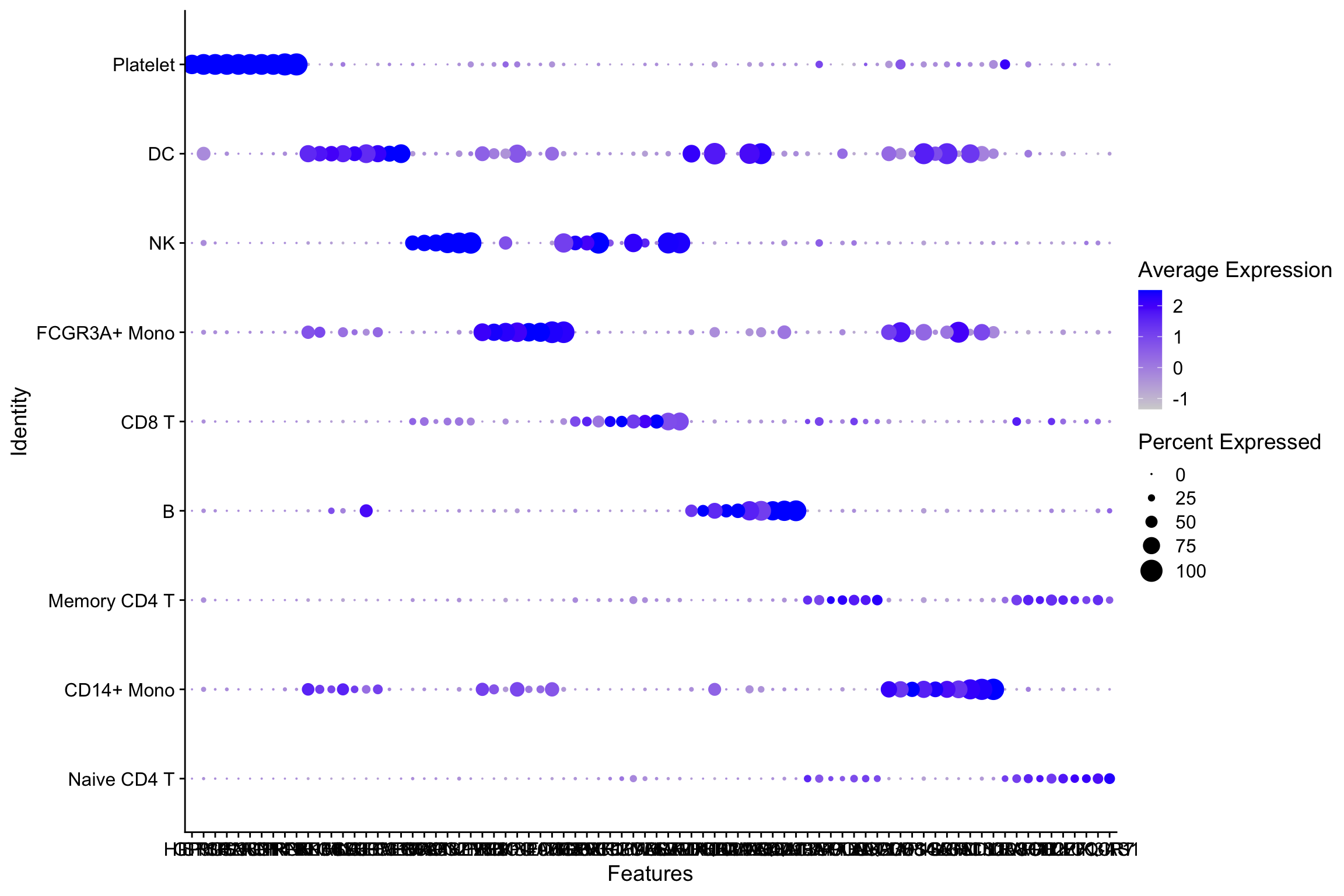### Let’s reproduce it using Complexheatmap

df<- p$data head(df) ## avg.exp pct.exp features.plot id avg.exp.scaled ## CCR7 2.993317 43.41534 CCR7 Naive CD4 T 2.337223 ## PIK3IP1 2.674179 42.11288 PIK3IP1 Naive CD4 T 1.844182 ## PRKCQ-AS1 2.167078 33.28509 PRKCQ-AS1 Naive CD4 T 2.123716 ## LEF1 2.003597 31.83792 LEF1 Naive CD4 T 2.027023 ## TCF7 2.253631 37.48191 TCF7 Naive CD4 T 1.773285 ## CD27 2.326533 39.07381 CD27 Naive CD4 T 1.210923 ### the matrix for the scaled expression exp_mat<-df %>% select(-pct.exp, -avg.exp) %>% pivot_wider(names_from = id, values_from = avg.exp.scaled) %>% as.data.frame() row.names(exp_mat) <- exp_mat$features.plot
exp_mat <- exp_mat[,-1] %>% as.matrix()

head(exp_mat)
##           Naive CD4 T          B Memory CD4 T FCGR3A+ Mono         NK
## CCR7         2.337223  0.4065916    0.6473101   -0.5357480 -0.6116367
## PIK3IP1      1.844182 -0.2277953    1.4179422   -0.6531667 -0.1884663
## PRKCQ-AS1    2.123716 -0.6830185    1.0323842   -0.5907663  0.0349381
## LEF1         2.027023 -0.5746819    1.4350437   -0.5707971 -0.4876044
## TCF7         1.773285 -0.4479782    1.5832831   -0.5337908 -0.6645233
## CD27         1.210923 -0.2029260    1.3852365   -0.7374133 -0.6415774
##                CD8 T CD14+ Mono         DC   Platelet
## CCR7      -0.4272161 -0.5827381 -0.5508353 -0.6829502
## PIK3IP1    0.2223521 -0.8180393 -0.9991901 -0.5978184
## PRKCQ-AS1  0.2375921 -0.6732668 -0.7407892 -0.7407892
## LEF1      -0.3271094 -0.4091405 -0.7288104 -0.3639229
## TCF7       0.2798941 -0.5843243 -0.6207981 -0.7850477
## CD27       1.3328521 -0.7261509 -0.7919650 -0.8289795
## the matrix for the percentage of cells express a gene

percent_mat<-df %>%
select(-avg.exp, -avg.exp.scaled) %>%
pivot_wider(names_from = id, values_from = pct.exp) %>%
as.data.frame()

row.names(percent_mat) <- percent_mat\$features.plot
percent_mat <- percent_mat[,-1] %>% as.matrix()

head(percent_mat)
##           Naive CD4 T         B Memory CD4 T FCGR3A+ Mono        NK     CD8 T
## CCR7         43.41534 15.759312     26.37131     5.095541  2.702703  4.747774
## PIK3IP1      42.11288 13.180516     40.92827    12.738854 13.513514 18.694362
## PRKCQ-AS1    33.28509  1.146132     29.53586     4.458599 11.486486 13.353116
## LEF1         31.83792  2.578797     32.27848     3.821656  4.054054  5.341246
## TCF7         37.48191  9.169054     36.91983    17.197452  8.108108 18.991098
## CD27         39.07381 12.034384     45.14768     4.458599  4.729730 25.816024
##           CD14+ Mono        DC Platelet
## CCR7        2.434077  8.333333 0.000000
## PIK3IP1     6.693712  2.777778 6.666667
## PRKCQ-AS1   1.419878  0.000000 0.000000
## LEF1        5.070994  0.000000 6.666667
## TCF7        9.736308 16.666667 6.666667
## CD27        3.042596  2.777778 0.000000
## the range is from 0 - 100
range(percent_mat)
##    0 100
## these two matrix have the same dimension
dim(exp_mat)
##  80  9
dim(percent_mat)
##  80  9
library(viridis)
library(Polychrome)

Polychrome::swatch(viridis(20))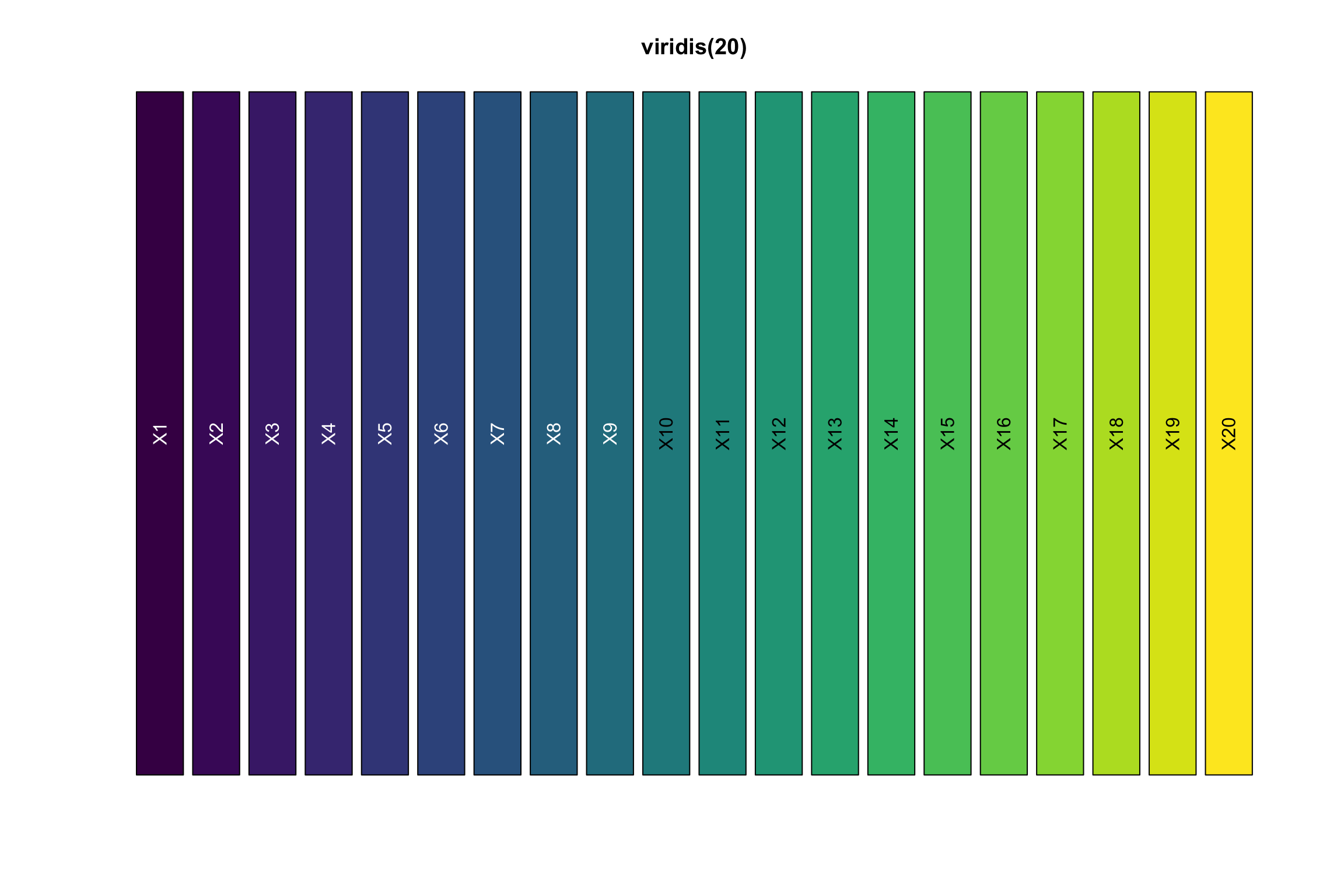## get an idea of the ranges of the matrix
quantile(exp_mat, c(0.1, 0.5, 0.9, 0.99))
##        10%        50%        90%        99%
## -0.6695752 -0.3567907  1.7156356  2.5000000
## any value that is greater than 2 will be mapped to yellow
col_fun = circlize::colorRamp2(c(-1, 0, 2), viridis(20)[c(1,10, 20)])

cell_fun = function(j, i, x, y, w, h, fill){
grid.rect(x = x, y = y, width = w, height = h,
gp = gpar(col = NA, fill = NA))
grid.circle(x=x,y=y,r= percent_mat[i, j]/100 * min(unit.c(w, h)),
gp = gpar(fill = col_fun(exp_mat[i, j]), col = NA))}
## also do a kmeans clustering for the genes with k = 4
Heatmap(exp_mat,
heatmap_legend_param=list(title="expression"),
column_title = "clustered dotplot",
col=col_fun,
rect_gp = gpar(type = "none"),
cell_fun = cell_fun,
row_names_gp = gpar(fontsize = 5),
row_km = 4,
border = "black")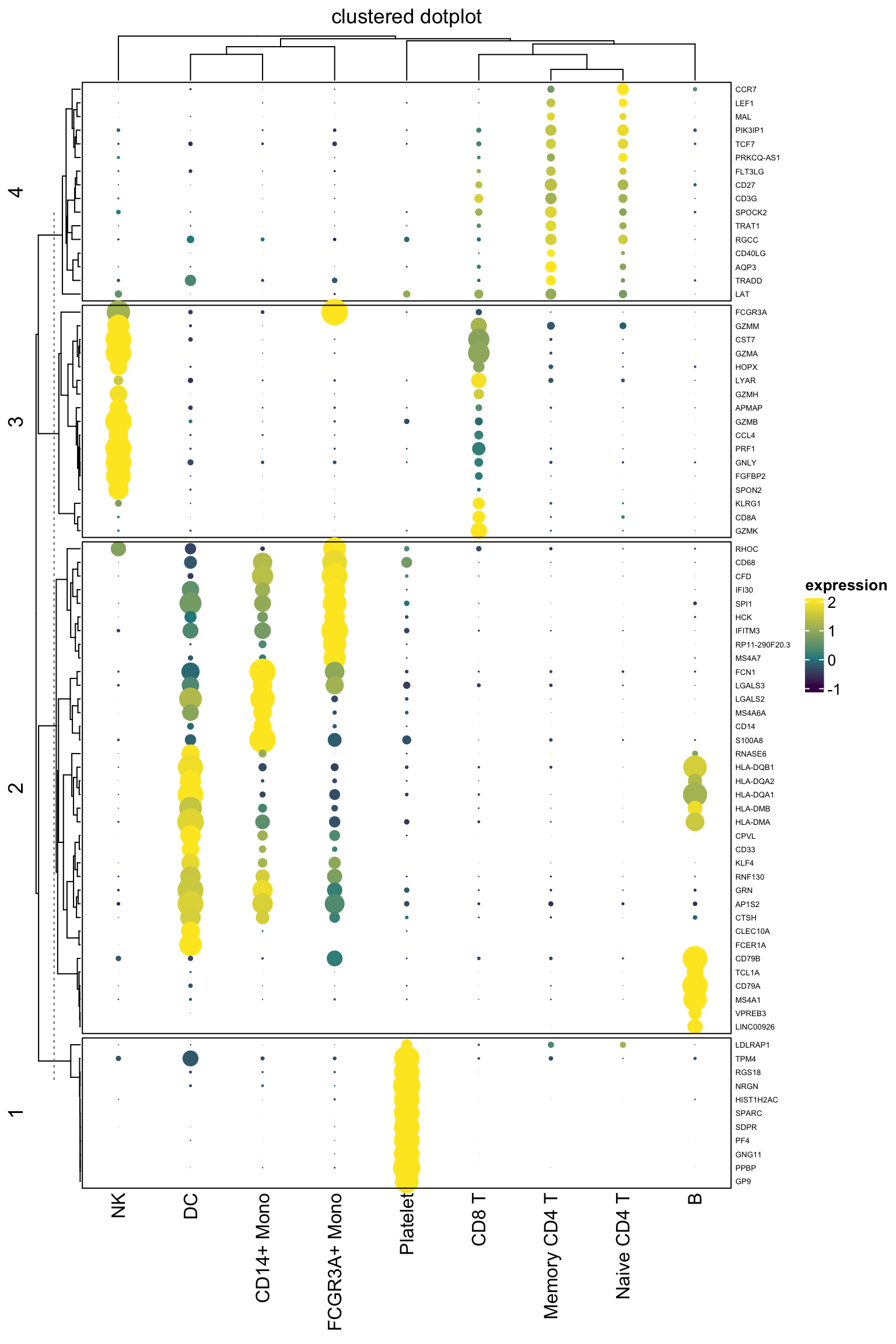It looks pretty decent. One can now take advantage of all the awesome annotation function to add annotation bars in complexheatmap.

colnames(exp_mat)
##  "Naive CD4 T"  "B"            "Memory CD4 T" "FCGR3A+ Mono" "NK"
##  "CD8 T"        "CD14+ Mono"   "DC"           "Platelet"
library(RColorBrewer)
cluster_anno<-  c("CD4T", "B", "CD4T", "Mono", "NK", "CD8T", "CD14_Mono", "DC", "Platelet")

column_ha<- HeatmapAnnotation(
cluster_anno = cluster_anno,
col = list(cluster_anno = setNames(brewer.pal(8, "Paired"), unique(cluster_anno))
),
na_col = "grey"
)

Heatmap(exp_mat,
heatmap_legend_param=list(title="expression"),
column_title = "clustered dotplot",
col=col_fun,
rect_gp = gpar(type = "none"),
cell_fun = cell_fun,
row_names_gp = gpar(fontsize = 5),
row_km = 4,
border = "black",
top_annotation = column_ha)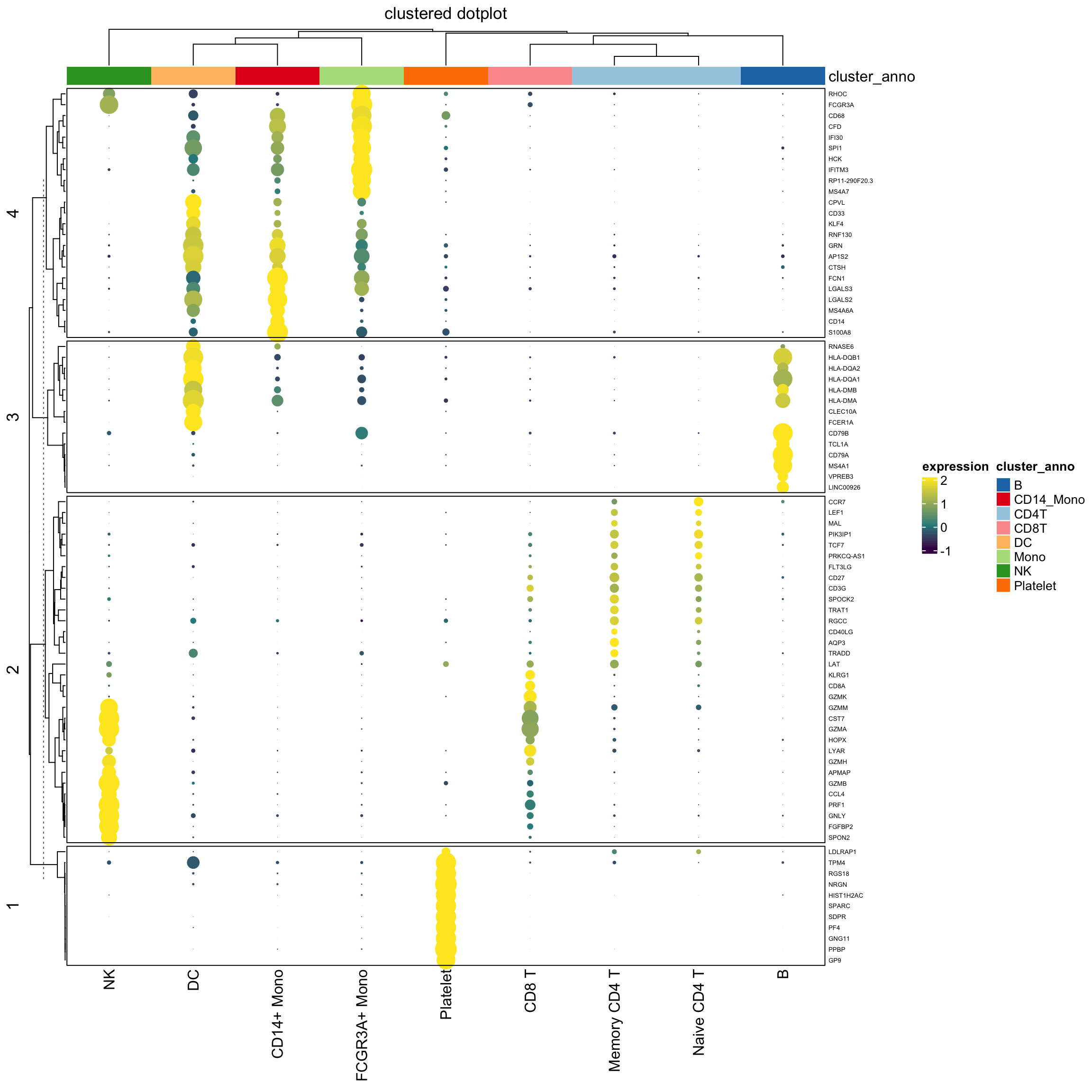### UPDATE

Alternatively you can use layer_fun which is a vectorized version of cell_fun. It will speed up plotting in interactive graphics devices. Just change percent_mat[i, j] to pindex(percent_mat, i, j) and keep other code unchanged, and assign to layer_fun argument.

One can also create a legend for the dot size for the percentages. https://jokergoo.github.io/ComplexHeatmap-reference/book/legends.html#add-customized-legends

I think for points, it might be the diameter. size is directly passed to grid.points() so it should mean the size of an arbitrary symbol.

Later, he confirmed that the size of points generated by grid.points(size = 10) is different from that from grid.circle(r=5)

For your case, I would suggest to also use grid.points in your layer_fun. If you use grid.circle in layer_fun, then you also need to use grid.circle to construct legend, which is a little bit complicated. You need to set graphics argument in Legend()

See the last example in Section 5.2. https://jokergoo.github.io/ComplexHeatmap-reference/book/legends.html#discrete-legends

### use grid.circle() in both the heatmap body and the legend

## To make the size of the dot in the heatmap body comparable to the legend, I used fixed
## size unit.(2, "mm) rather than min(unit.c(w, h).

layer_fun = function(j, i, x, y, w, h, fill){
grid.rect(x = x, y = y, width = w, height = h,
gp = gpar(col = NA, fill = NA))
grid.circle(x=x,y=y,r= pindex(percent_mat, i, j)/100 * unit(2, "mm"),
gp = gpar(fill = col_fun(pindex(exp_mat, i, j)), col = NA))}

lgd_list = list(
Legend( labels = c(0,0.25,0.5,0.75,1), title = "pt",
graphics = list(
function(x, y, w, h) grid.circle(x = x, y = y, r = 0 * unit(2, "mm"),
gp = gpar(fill = "black")),
function(x, y, w, h) grid.circle(x = x, y = y, r = 0.25 * unit(2, "mm"),
gp = gpar(fill = "black")),
function(x, y, w, h) grid.circle(x = x, y = y, r = 0.5 * unit(2, "mm"),
gp = gpar(fill = "black")),
function(x, y, w, h) grid.circle(x = x, y = y, r = 0.75 * unit(2, "mm"),
gp = gpar(fill = "black")),
function(x, y, w, h) grid.circle(x = x, y = y, r = 1 * unit(2, "mm"),
gp = gpar(fill = "black")))
))

set.seed(123)
hp<- Heatmap(exp_mat,
heatmap_legend_param=list(title="expression"),
column_title = "clustered dotplot",
col=col_fun,
rect_gp = gpar(type = "none"),
layer_fun = layer_fun,
row_names_gp = gpar(fontsize = 5),
row_km = 4,
border = "black",
top_annotation = column_ha)

draw( hp, annotation_legend_list = lgd_list)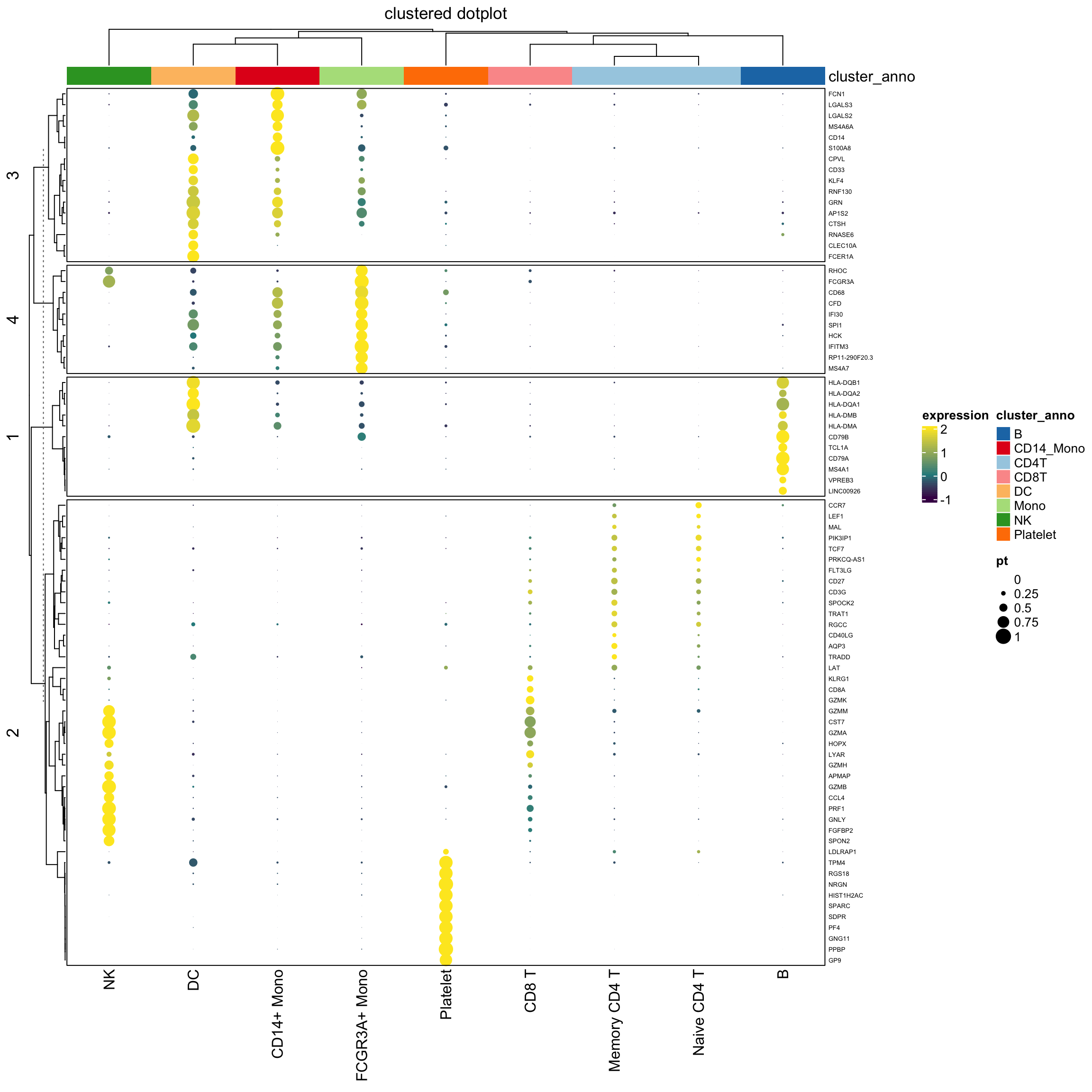Note that, we use the percentage as the radius of the circle. The area of the dot is pi *r^2. It will appear 4 times different if the original percentage is 0.5 versus 1 (2 times difference) which may not be desirable. see https://stackoverflow.com/questions/64067450/does-size-for-ggplot2geom-point-refer-to-radius-diameter-area-or-somethin

We can do a square root of the radius:

layer_fun1 = function(j, i, x, y, w, h, fill){
grid.rect(x = x, y = y, width = w, height = h,
gp = gpar(col = NA, fill = NA))
grid.circle(x=x,y=y,r= sqrt(pindex(percent_mat, i, j)/100)  * unit(2, "mm"),
gp = gpar(fill = col_fun(pindex(exp_mat, i, j)), col = NA))}

lgd_list1 = list(
Legend( labels = c(0,0.25,0.5,0.75,1), title = "pt",
graphics = list(
function(x, y, w, h) grid.circle(x = x, y = y, r = 0  * unit(2, "mm"),
gp = gpar(fill = "black")),
function(x, y, w, h) grid.circle(x = x, y = y, r = sqrt(0.25) * unit(2, "mm"),
gp = gpar(fill = "black")),
function(x, y, w, h) grid.circle(x = x, y = y, r = sqrt(0.5) * unit(2, "mm"),
gp = gpar(fill = "black")),
function(x, y, w, h) grid.circle(x = x, y = y, r = sqrt(0.75) * unit(2, "mm"),
gp = gpar(fill = "black")),
function(x, y, w, h) grid.circle(x = x, y = y, r = 1 * unit(2, "mm"),
gp = gpar(fill = "black")))
))

set.seed(123)
hp1<- Heatmap(exp_mat,
heatmap_legend_param=list(title="expression"),
column_title = "clustered dotplot",
col=col_fun,
rect_gp = gpar(type = "none"),
layer_fun = layer_fun1,
row_names_gp = gpar(fontsize = 5),
row_km = 4,
border = "black",
top_annotation = column_ha)

draw( hp1, annotation_legend_list = lgd_list1)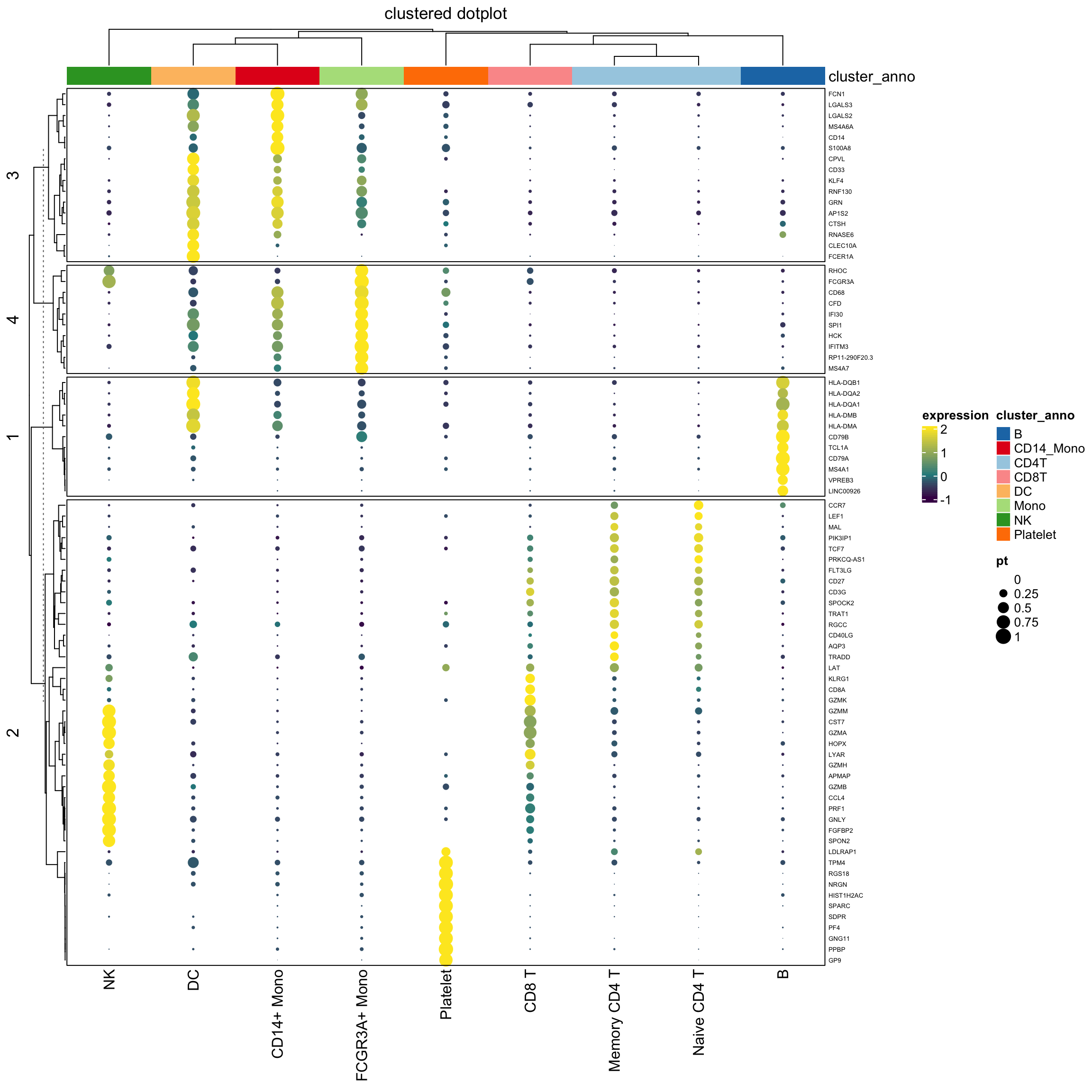### use grid.points() in both the heatmap body and the legend

## note for grid.points, use col for the filling color of the points, while in grid.circle, use fill for the filling color of the circle. I should learn more about {grid}

layer_fun2 = function(j, i, x, y, w, h, fill){
grid.rect(x = x, y = y, width = w, height = h,
gp = gpar(col = NA, fill = NA))
grid.points(x = x, y = y,
gp = gpar(col = col_fun(pindex(exp_mat, i, j))),
size = pindex(percent_mat, i, j)/100 * unit(4, "mm"),
pch = 16
)
}

lgd_list2 = list(
Legend( labels = c(0,0.25,0.5,0.75,1), title = "pt", type = "points", pch = 16, size = c(0,0.25,0.5,0.75,1) * unit(4,"mm"),
legend_gp = gpar(col = "black")))

set.seed(123)
hp2<- Heatmap(exp_mat,
heatmap_legend_param=list(title="expression"),
column_title = "clustered dotplot",
col=col_fun,
rect_gp = gpar(type = "none"),
layer_fun = layer_fun2,
row_names_gp = gpar(fontsize = 5),
row_km = 4,
border = "black",
top_annotation = column_ha)

draw( hp2, annotation_legend_list = lgd_list2)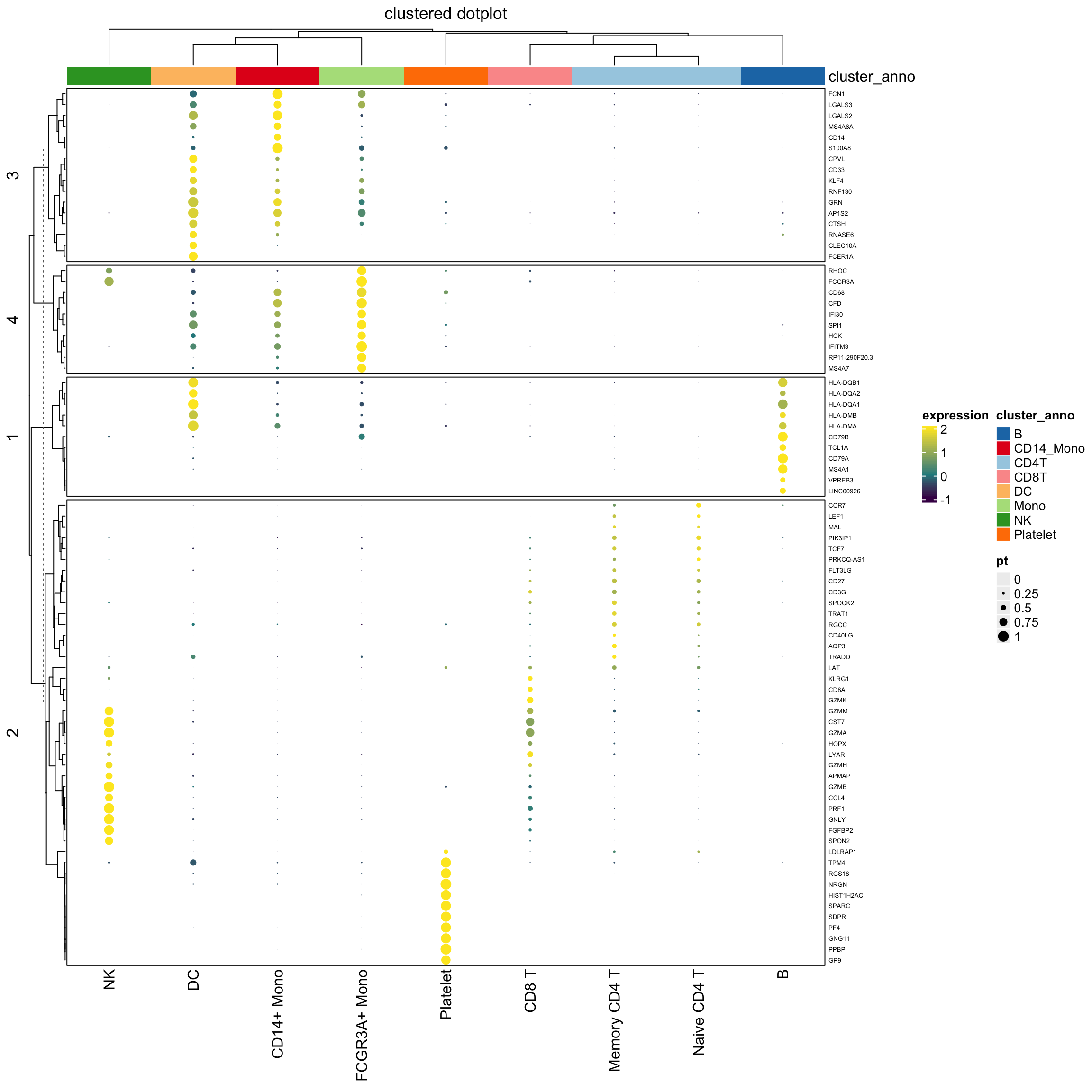### Conclusion

I would use grid.circle to draw the dots in the legend and the heatmap body since I can fine-tune the looking of the dot size. I am not entirely sure how the size argument determines the size of the dots in grid.points(size).

Again, thanks Zuguang Gu for this awesome package!!